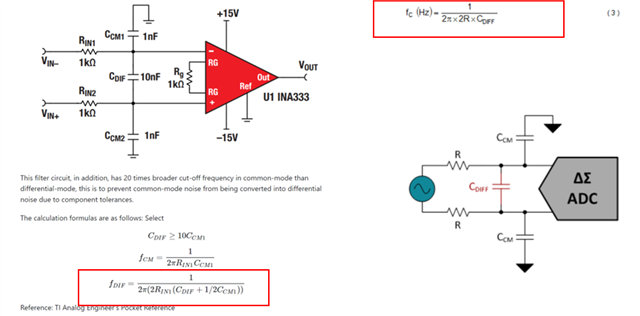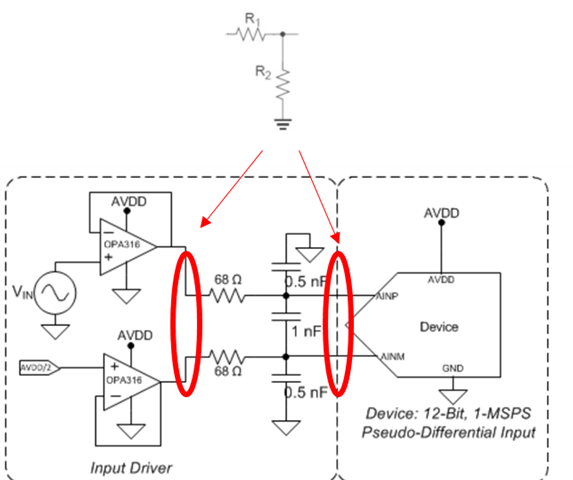If you have a related question, please click the "Ask a related question" button in the top right corner. The newly created question will be automatically linked to this question.

# ADS131M08: common mode fc? & other....

Hi~. I have three questions

1) In the Ti site, there are two diff fc calculation equations as below.  Which one is right?2) I need to use a voltage divider resistor to lower the adc input value. If the divider resistor is in front or behind the filter circuit, or if the without an additional filter, connect the pull-down resistor in parallel to common noise filter cap Ccm to make the voltage divider and the filter together, which is more effective?3) ) What is the reason for common mode filter when using diff adc? If common noise occurs in AINP&AINN, does it become AINP-AINN and noise is removed?

Thanks.

• Hello Kwon,

Thanks for posting on the forum!

Let me talk with the team and get back with you within 3 or 4 days.

Thanks,

-Cole

• Hello Kwon,

1) In the Ti site, there are two diff fc calculation equations as below.  Which one is right?

Part of me wants to let you derive it. Assuming you have a background or education in electronics, Kirchhoff's circuit laws can get you a lot of the way there. The practical answer is they are both correct but the technical answer is that equation that contains the C_CM/2 is more correct.

You will often see engineers follow some rules of thumb that say "if a factor is a degree of magnitude (or 10 times smaller) then it doesn't exist (or realistically contribute)". This is what is happening. If we substitute the rule that C_diff/10 > C_CM, we get (C_diff + C_diff/20) = C_diff*21/20. 21/20 is 1.05 which means that it contributes less than 5% of where the cut-off frequency. The anti-aliasing filter's purpose is to attenuate aliasing signals and high frequency differential noise. When you do the math, the 5% would be 0.5dB difference, why does 0.5dB matter when we're at -40dB attenuation? This is the type of thought process is going through an engineer's head.

A lot of these calculations are rough estimates anyways. Simulations will move it a bit closer but resistor tolerances are the real world. So, the engineer's job is to get "close" in the context. There might be very specific less than 0.1% accuracy numbers for filtering which will require some precise equations and simulations, but an anti-aliasing filter very rarely have these kinds of specifications. Hope that makes sense.

2) I need to use a voltage divider resistor to lower the adc input value. If the divider resistor is in front or behind the filter circuit, or if the without an additional filter, connect the pull-down resistor in parallel to common noise filter cap Ccm to make the voltage divider and the filter together, which is more effective?

I would put all resistor dividers as close to the signal source as you can. Hopefully the divider is selected in a way that brings the min and max voltage within AVDD or the buffer (or voltage follower) specifications. The buffer's purpose is to keep a low impedance node looking into the input of the PGA (and ADC). There's a lot of reasons for this, and I encourage you to check out the TI Precision Labs video series I linked below if don't know why. Specifically the stability section.

3) ) What is the reason for common mode filter when using diff adc? If common noise occurs in AINP&AINN, does it become AINP-AINN and noise is removed?

This question relates to the common mode rejection ratio (CMRR) and common mode offset error. There's a really good TI Precision Labs video I recommend you check out: https://training.ti.com/ti-precision-labs-op-amps-common-mode-rejection . It might be for op amps but an ADC (and the ADS131M08 in this case) has an integrated PGA which is just an op amp at the first input stage.

In summary, if you apply a common mode voltage to the input of the PGA, a different offset voltage comes out and this is essentially an error in the measurement. Adding filtering ensure that the common mode voltage will remain around a constant value so no higher frequency noise starts to affect the common mode voltage. In other words, if the common mode voltage oscillates then the error is not a constant and will also oscillate. DC error is much easier to calibrate for than an oscillating error (AC error).

Best,

-Cole